How Cheenta works to ensure student success?
Explore the Back-Story

# Test of Mathematics Solution Subjective 35 - Divisibility by 16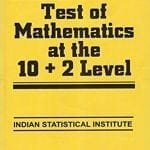Test of Mathematics Solution Subjective 35 (from ISI Entrance). The book, Test of Mathematics at 10+2 Level is Published by East West Press. This problem book is indispensable for the preparation of I.S.I. B.Stat and B.Math Entrance.

## Problem

(a) Prove that, for any odd integer n,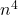when divided by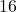always leaves remainder.

(b) Hence or otherwise show that we cannot find integers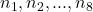such that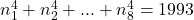.

## Solution

For part (a) we consider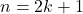and expand it's fourth power binomially to get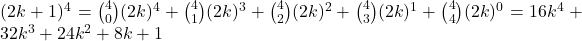Now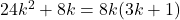; if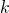is even then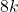is divisible byand ifis odd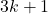is even and product ofandis divisible by. Since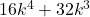is already divisible bywe conclude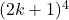when divided bygivesas remainder.

For part (b) we note thatwhen divided by, produces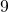as the remainder. Each of the eight of fourth powers when divided byproduces either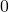(when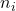is even) or(whenis odd using part (a)) as remainder. Thus they can add up to at most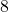(modulo) hence can never be equal to(whichis modulo).Test of Mathematics Solution Subjective 35 (from ISI Entrance). The book, Test of Mathematics at 10+2 Level is Published by East West Press. This problem book is indispensable for the preparation of I.S.I. B.Stat and B.Math Entrance.

## Problem

(a) Prove that, for any odd integer n,when divided byalways leaves remainder.

(b) Hence or otherwise show that we cannot find integerssuch that.

## Solution

For part (a) we considerand expand it's fourth power binomially to getNow; ifis even thenis divisible byand ifis oddis even and product ofandis divisible by. Sinceis already divisible bywe concludewhen divided bygivesas remainder.

For part (b) we note thatwhen divided by, producesas the remainder. Each of the eight of fourth powers when divided byproduces either(whenis even) or(whenis odd using part (a)) as remainder. Thus they can add up to at most(modulo) hence can never be equal to(whichis modulo).

This site uses Akismet to reduce spam. Learn how your comment data is processed.

### Knowledge Partner